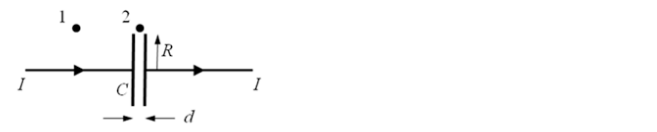# Problem: A constant current I is supplied for a brief time to charge a parallel plate capacitor. The capacitor has circular plates of radius R with gap d (d &lt;&lt; R). Point 1 is at R + d from the wire, and point 2, is at a distance R + d from the center of the capacitor. During the time interval that the constant current I is flowing through the capacitor, the magnetic field at point 2, B2 is: a) B2 &gt; B1 b) B2 = B1 c) 0 &lt; B2 &lt; B1 d) B2 = 0 e) not enough information to tell

🤓 Based on our data, we think this question is relevant for Professor Romani's class at STANFORD.

###### Problem Details

A constant current I is supplied for a brief time to charge a parallel plate capacitor. The capacitor has circular plates of radius R with gap d (d << R). Point 1 is at R + d from the wire, and point 2, is at a distance R + d from the center of the capacitor.

During the time interval that the constant current I is flowing through the capacitor, the magnetic field at point 2, B2 is:

a) B2 > B1

b) B2 = B1

c) 0 < B2 < B1

d) B2 = 0

e) not enough information to tell